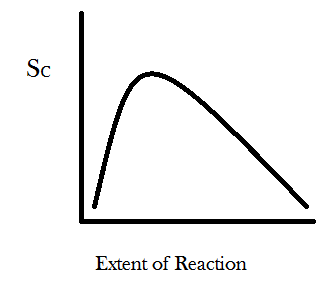# New Criticals

We can generalize this result further by imagining a new parameter called the “extent of reaction.” In the example where one begins with four A’s and four B’s then the reaction A + B → C can happen, at most, four times. The least it can happen is zero times. In a more realistic scenario there would be something like 10^26 molecules in your system and the reaction might occur or not many times. So we graph the extent of reaction as a percent of the total times the reaction can possibly occur and let it range between 0 and 100. You can take the W number calculated from the permutational equation for each time the reaction might occur and stick it into Boltzmann’s entropy formula, S = k*log(W), and then graph the entropy S as function of how many times the reaction has occurred, with the “extent of reaction” parameter on the x-axis and configurational entropy on the y-axis. You get something that looks like this:For the simple reaction A + B → C the entropy is maximized at about 29% completion. For different reactions with different ratios of reactants to products the configurational entropy will be maximized at different percents of completion. Also keep in mind that the systems true equilibrium (maximum entropy) can only be found by taking into account the change in the number of energetic microstates as well. The point  I would like you to take away from this graph is that the slope of the curve is always positive for small values of the extent of reaction and always negative for large values. The physical meaning of these asymptotic slopes is that matter-randomization considerations promote all chemical transformations through some compositional range. Undergraduate chemistry students are taught this lesson as a particular instance of “Le Chatelier’s Principle.”

One way of explaining this principle of chemical reactions is saying that if you build up a large enough concentration of one kind of thing in one place, even energetically unfavorable processes will start to occur spontaneously as a way of equalizing this improbability in the distribution of matter. Crucially for evolution, these considerations always promote the formation of something new, although how much of the new thing gets made will depend upon the energetics of the process (this basically amounts to how much heat the reaction gives off or requires).

Matter-randomization is also the basis for “Murphy’s law,” the unofficial, cynical corollary of the second law. Do we see now that the real issue isn’t that "whatever can go wrong will go wrong," but rather that thermodynamics guarantees that anything that can happen must happen to some extent. Conversely, no chemical process ever proceeds entirely to completion. That's because what's true for the forward reaction is also true for the back reaction. Or just imagine that we start off our previous example with a container holding only four molecules of "C." We'd be starting on the far right side of the above graph. The way to increase the microstates from this initial state is to have C's break apart back into A's and B's.  These assertions requires strong qualification though.